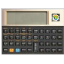# Simplexety

September 18th, 2020 - Free - 100% Safe(2 votes, average: 5.00 out of 5)
• Review

If you constantly perform and use mathematical expressions, then you simply must know about Simplexety. It’s one of the best but also one of the feature-rich applications when it comes to editing and creating mathematical expressions through the VBScript engine. If you’re a mathematics teacher, student, or engineer, then you must know this tool is much more than a scientific calculator.

### A Feature-Rich Application

Simplexety is a very comprehensive application with a lot of different functions and features. When you first use the app, the interface might seem a little complex but worry not: with some learning and some time using the app, you’ll quickly get to grips with it. There are numerous symbols and mathematical expressions in the interface that can seem complicated at first.

But once you’re used to the interface, you’ll start to learn just how effective and useful it is. You can perform several mathematical calculations and operations, including multiplication, addition, division, multiplication, and many other more complex functions, such as trigonometric, polar, and parametric functions.

### Use Well-Known Mathematical Theories and Formulas

This application allows you to use all of the most well-known mathematical theories and formulas to your advantage. These can be found in science books, but you have them collected here in one nicely organized place.

Depending on what you need from the app, you can use and combine the most popular formulas where you can simply edit your calculations and make changes at any time you want. You can also use Greek symbols, but note that you might need to double-click each symbol before using it. Then you can simply copy the generated results in a clipboard and use them for the next time.

Also, the application lets you keep track of the time and set several time parameters, which makes using the app much more fun but also more effective. You can use the most common measurement units for trigonometric functions, or you can create other custom functions and constants.

There’s also a built-in parser that allows you to perform various calculations and formulas with the help of time settings, including determining the current year, week, day, moon phase, or even season or epoch. In addition, there’s the Graph function that allows you to display each equation you make.

### Conclusion

Simplexety is one of the most complete and complex mathematical apps that allows you to use all of the most common formulas and calculations in one place. It has some very complex mathematical functions included, so it might take some time to get used to it. But once you get to know it closely, you’ll learn just how powerful it can be for creating custom and editing mathematical formulas you’ve made.

## Technical details

8.6.0.0
File name:
Simplexety.zip
MD5 checksum:
2A269769C09A1F9D9342EA2EC405BBAF
File size:
3.06 MB